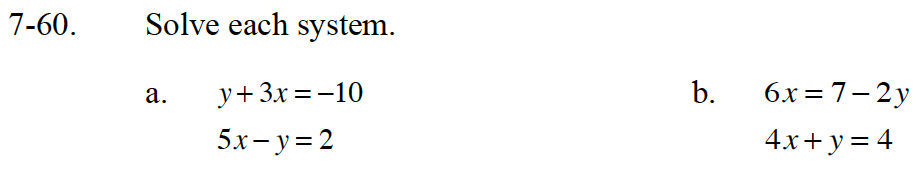Home > CC4 > Chapter 7 > Lesson 7.1.5 > Problem7-60

7-60.
1. Solve each system. Homework Help ✎

1. y + 3x = −10
5xy = 2

2. 6x = 7 − 2y
4x
+ y = 4The second equation can be rewritten as −y + 5x = 2 (Commutative Property of Addition).

(−1, −7)

Which equation should you change into y = mx + b form so that you can substitute it into the other equation?